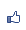Contents ...
udn網路城邦
[Excel VBA] 九宮格智慧盤遊戲程式原理(下)
2009/03/14 19:39

Private Sub x1_Click()
BlockRoutine
End Sub

Sub BlockRoutine()  '方塊按鈕要做的事
If StartGame Then            '若game已開始就
ThisBtn = Right(ActiveControl.Name, 1) '取得名稱最右的一個字元
Call SwapBlank(ThisBtn) '看看要不要交換?
If IsDone Then Call StopGame      '若已完成，就停止遊戲
End If
End Sub

SwapBlank() 從 BlockRoutine() 傳給它的 "按鈕的名稱最右的一個字元" (參數 BtnNo)，就可以得到 (被點到的這個) 按鈕方塊上的數字，請對照底下的程式碼，就是 Controls("x" & BtnNo).Caption，沒有問題。

SwapBlank() 要將 (被點到的這個) 按鈕方塊上的數字，與相鄰的空白方塊按鈕交換。它必須去檢查 "相鄰的" 按鈕方塊哪一個的 Caption 是空白的 (如果它自己的 Caption 是空白，就不需去檢查相鄰的)，那誰是與它相鄰的呢？

Neighbors(5)(1) --> "2" --> x2
Neighbors(5)(2) --> "4" --> x4
Neighbors(5)(3) --> "6" --> x6
Neighbors(5)(4) --> "8" --> x8

*註：因為我的 Userform1 程式碼開頭第一列寫了 Option Base 1，所以陣列的最小註標 (index) 是從 1 開始。

Sub SwapBlank(BtnNo As Variant)
Dim i As Integer
Dim Neighbors

Neighbors = Array(Array("2", "4"), _
Array("1", "3", "5"), _
Array("2", "6"), _
Array("1", "5", "7"), _
Array("2", "4", "6", "8"), _
Array("3", "5", "9"), _
Array("4", "8"), _
Array("5", "7", "9"), _
Array("6", "8"))

If Controls("x" & BtnNo).Caption <> "" Then '若這不是空白方塊按鈕, 就
For i = 1 To UBound(Neighbors(BtnNo))   '檢查每個相鄰方塊按鈕上的數字
If Controls("x" & Neighbors(BtnNo)(i)).Caption = "" Then '若是鄰居是空白
Controls("x" & Neighbors(BtnNo)(i)).Caption = Controls("x" & BtnNo).Caption '就把數字給它
Controls("x" & BtnNo).Caption = ""                   '然後將此按鈕的顯示設為空白
Moves = Moves + 1            '移動次數增加一次
Label2.Caption = CStr(Moves) '更新移動次數顯示
Exit For            '已完成交換就可以跳出迴圈
End If
Next i
End If
End Sub

BlockRoutine() 呼叫 SwapBlank() 之後，就會呼叫 IsDone()，如果九個方塊上的數字都回到原位了，IsDone() 會傳回 True。九個方塊上的數字都回到原位，就是說：x1 上面的數字應  1, x2 上面的數字為 2,......依此類推：

Function IsDone() As Boolean
Dim i As Integer

IsDone = True
For i = 1 To 8
If Val(Controls("x" & i).Caption) <> i Then IsDone = False
Next i
End Function1樓.
2010/01/02 21:59